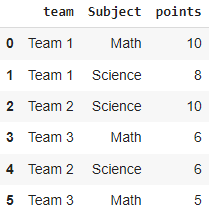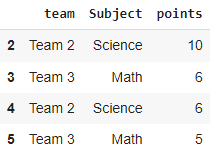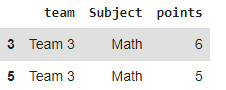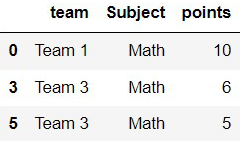Open in App
Not now

# How to Drop Rows that Contain a Specific String in Pandas?

• Difficulty Level : Basic
• Last Updated : 03 Dec, 2021

In this article, we are going to see how to drop rows that contain a specific string in pandas. Now, to drop the rows with a specific string we can use the contains() function from the pandas library.

Syntax: series.str.contains(string, case=True, flags=0, na=None, regex=True)

Returns  –

Series or index of Boolean Values

Basically, this function will search for the string in the given column and returns the rows respective to that. For this, we need to create a new data frame by filtering the data frame using this function.

Syntax:

df[ df[ “column” ].str.contains( “someString” )==False ]

Example: Create DataFrame

## Python3

 `# Importing the library ` `import` `pandas as pd ` ` `  `# Dataframe ` `df ``=` `pd.DataFrame({``'team'``: [``'Team 1'``, ``'Team 1'``, ``'Team 2'``, ` `                            ``'Team 3'``, ``'Team 2'``, ``'Team 3'``], ` `                   ``'Subject'``: [``'Math'``, ``'Science'``, ``'Science'``, ` `                               ``'Math'``, ``'Science'``, ``'Math'``], ` `                   ``'points'``: [``10``, ``8``, ``10``, ``6``, ``6``, ``5``]}) ` ` `  `# display ` `df `

Output:## Method 1: Dropping the rows that contain a specific string

In this method, we are going to find the rows with str.contains() function which will basically take the string from the series and check for the match of the given string, and using a boolean we are selecting the rows and setting them to False will help us to neglect the selected rows and keep the remaining rows.

syntax:  df[df[“column_name”].str.contains(“string”)==False]

Example:

In the following example, we are going to select all the teams except  “Team 1”.

## Python3

 `# importing the library ` `import` `pandas as pd ` ` `  `# Dataframe ` `df ``=` `pd.DataFrame({``'team'``: [``'Team 1'``, ``'Team 1'``, ``'Team 2'``, ` `                            ``'Team 3'``, ``'Team 2'``, ``'Team 3'``], ` `                   ``'Subject'``: [``'Math'``, ``'Science'``, ``'Science'``,  ` `                               ``'Math'``, ``'Science'``, ``'Math'``], ` `                   ``'points'``: [``10``, ``8``, ``10``, ``6``, ``6``, ``5``]}) ` ` `  `# Dropping the team 1 ` `df ``=` `df[df[``"team"``].``str``.contains(``"Team 1"``) ``=``=` `False``] ` ` `  `df `

Output## Method 2: Dropping the rows with more than one string

Same as method 1, we follow the same steps here but with a bitwise or operator to add an extra string to search for.

syntax: df = df[df[“column_name”].str.contains(“string1|string2”)==False]

Example:

In the following, program we are going to drop the rows that contain “Team 1” or “Team 2”.

## Python3

 `# importing the library ` `import` `pandas as pd ` ` `  `# Dataframe ` `df ``=` `pd.DataFrame({``'team'``: [``'Team 1'``, ``'Team 1'``, ``'Team 2'``,  ` `                            ``'Team 3'``, ``'Team 2'``, ``'Team 3'``], ` `                   ``'Subject'``: [``'Math'``, ``'Science'``, ``'Science'``,  ` `                               ``'Math'``, ``'Science'``, ``'Math'``], ` `                   ``'points'``: [``10``, ``8``, ``10``, ``6``, ``6``, ``5``]}) ` ` `  `# Dropping the rows of team 1 and team 2 ` `df ``=` `df[df[``"team"``].``str``.contains(``"Team 1|Team 2"``) ``=``=` `False``] ` ` `  `# display ` `df `

Output:## Method 3: Drop rows with the given partial string

Here we are using the same function with a join method that carries the part of the word we need to search.

syntax:

df[ ~df.column_name.str.contains(‘|’.join([“string”])) ]

Example:

In this following program, the situation is different from the above two cases. Here we are going to select and drop the rows with the given partial string. For example, we are going to drop the rows with “Sci” on the column subjects.

## Python3

 `# importing the library ` `import` `pandas as pd ` ` `  `# Dataframe ` `df ``=` `pd.DataFrame({``'team'``: [``'Team 1'``, ``'Team 1'``, ``'Team 2'``,  ` `                            ``'Team 3'``, ``'Team 2'``, ``'Team 3'``], ` `                   ``'Subject'``: [``'Math'``, ``'Science'``, ``'Science'``, ` `                               ``'Math'``, ``'Science'``, ``'Math'``], ` `                   ``'points'``: [``10``, ``8``, ``10``, ``6``, ``6``, ``5``]}) ` ` `  `# Dropping the rows with "Sci" ` `# identify partial string ` `discard ``=` `[``"Sci"``] ` ` `  `# drop rows that contain the partial string "Sci" ` `df[~df.Subject.``str``.contains(``'|'``.join(discard))] ` ` `  `#display ` `df`

Output:My Personal Notes arrow_drop_up
Related Articles